# [翻译] 使用 TensorFlow 进行分布式训练

## 1. 概述

Tf.distribute.Strategy 是一个可在多个 GPU、多台机器或 TPU 上进行分布式训练的 TensorFlow API。使用此 API，您只需改动较少代码就能基于现有模型和训练代码来实现单机多卡，多机多卡等情况的分布式训练。

tf.distribute.Strategy 旨在实现以下目标：

• 覆盖不同维度的用户用例。
• 易于使用，支持多种用户（包括研究人员和 ML 工程师等）。
• 提供开箱即用的高性能。
• 从用户模型代码之中解耦，这样可以轻松切换策略。
• 支持 Custom Training Loop，Estimator，Keras。
• 支持 eager excution。

Tf.distribute.Strategy 可用于 Keras，Model.fit等高级 API，也可用来分布自定义训练循环（以及（一般来说）使用 TensorFlow 的任何计算）。比如将模型进行构建和 model.compile() 调用封装在 strategy.scope() 内部。

## 2. 策略类型

Tf.distribute.Strategy 打算涵盖不同轴上的许多用例。目前已支持其中的部分组合，将来还会添加其他组合。其中一些轴包括：

• 同步和异步训练：这是通过数据并行进行分布式训练的两种常用方法。在同步训练中，所有工作进程都同步地对输入数据的不同片段进行训练，并且会在每一步中聚合梯度。在异步训练中，所有工作进程都独立训练输入数据并异步更新变量。通常情况下，同步训练通过全归约（all-reduce）实现，而异步训练通过参数服务器架构实现。
• 硬件平台：您可能需要将训练扩展到一台机器上的多个 GPU 或一个网络中的多台机器（每台机器拥有 0 个或多个 GPU），或扩展到 Cloud TPU 上。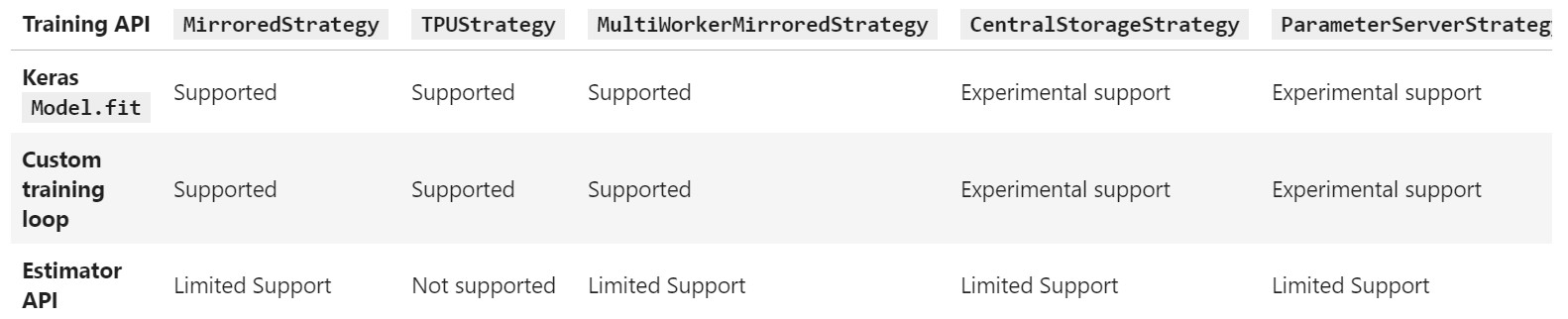### 2.1 MirroredStrategy

Tf.distribute.MirroredStrategy 支持在一台机器的多个 GPU 上进行同步分布式训练（单机多卡数据并行）。该策略会为每个 GPU 设备创建一个模型副本。模型中的每个变量都会在所有副本之间进行镜像。这些变量将共同形成一个名为 MirroredVariable 的概念上的单个变量。通过应用相同的更新，这些变量保持彼此保持同步。

MirroredVariable 的同步更新只是提高了计算速度，但并不能像 CPU 并行那样可以把内存之中的变量共享。即，显卡并行计算只是提高速度，并不会让用户数据量翻倍。增加数据仍然会抛出来内存溢出错误。

MirroredStrategy 使用高效的全归约（all-reduce）算法在设备之间传递变量更新。全归约（all-reduce）算法通过把各个设备上的张量加起来使其聚合，并使其在每个设备上可用。这是一种非常高效的融合算法，可以显著减少同步开销。根据设备之间可用的通信类型，可以使用的全归约（all-reduce）算法和实现方法有很多。默认使用 NVIDIA NCCL 作为全归约（all-reduce）实现。您可以选择我们提供的其他选项，也可以自己编写。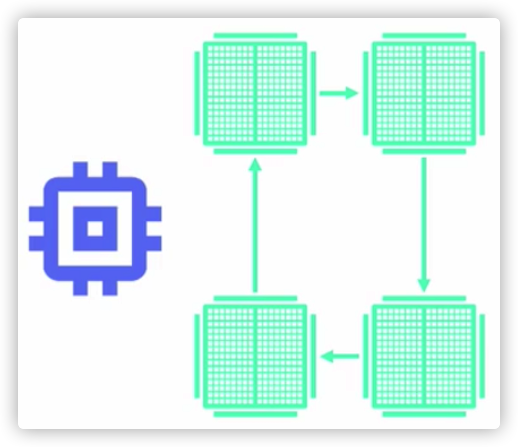``````mirrored_strategy = tf.distribute.MirroredStrategy()
``````

``````mirrored_strategy = tf.distribute.MirroredStrategy(devices=["/gpu:0", "/gpu:1"])
``````

``````mirrored_strategy = tf.distribute.MirroredStrategy(
cross_device_ops=tf.distribute.HierarchicalCopyAllReduce())
``````

### 2.2 TPUStrategy

``````cluster_resolver = tf.distribute.cluster_resolver.TPUClusterResolver(     tpu=tpu_address) tf.config.experimental_connect_to_cluster(cluster_resolver) tf.tpu.experimental.initialize_tpu_system(cluster_resolver) tpu_strategy = tf.distribute.experimental.TPUStrategy(cluster_resolver)
``````

TPUClusterResolver 实例可帮助定位 TPU。在 Colab 中，您无需为其指定任何参数。

• 在 tpu 参数中指定 TPU 资源的名称。
• 在程序开始时显式地初始化 TPU 系统。这是使用 TPU 进行计算前的必须步骤。初始化 TPU 系统还会清除 TPU 内存，所以为了避免丢失状态，请务必先完成此步骤。

### 2.3 MultiWorkerMirroredStrategy

Tf.distribute.experimental.MultiWorkerMirroredStrategy与 MirroredStrategy 非常相似。它实现了跨多个工作进程的同步分布式训练（多机多卡分布式版本），而每个工作进程可能有多个 GPU。与 MirroredStrategy 类似，它也会跨所有工作进程在每个设备的模型中创建所有变量的副本。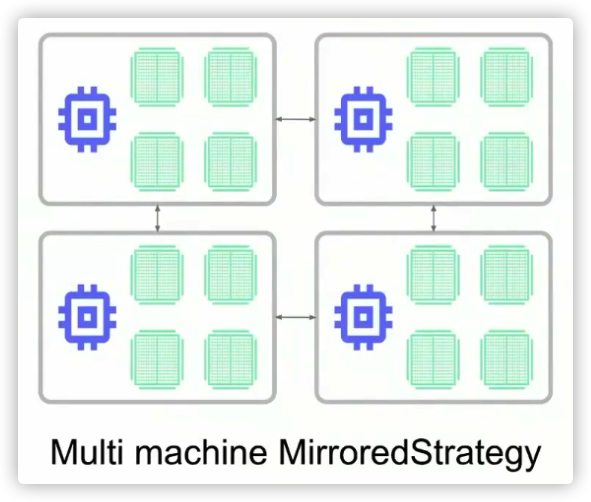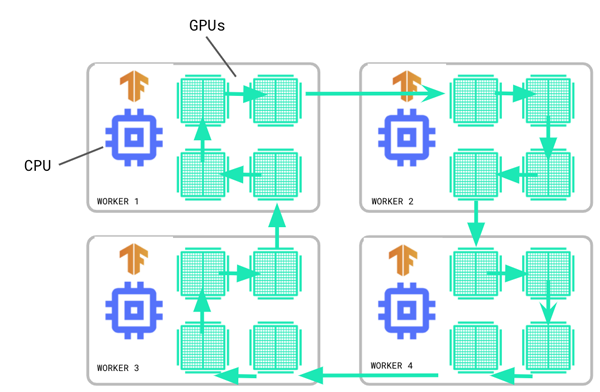``````multiworker_strategy = tf.distribute.experimental.MultiWorkerMirroredStrategy()
``````

MultiWorkerMirroredStrategy 目前为您提供两种不同的集合运算实现方法。CollectiveCommunication.RING通过将 RPC 用作通信层来实现基于环的集合，支持CPU和GPU。CollectiveCommunication.NCCL 使用 NCCL 来实现集合。CollectiveCommunication.AUTO 会将选择推迟到运行时。集合实现的最佳选择取决于 GPU 的数量和种类，以及集群中的网络互连。您可以通过以下方式来指定：

``````communication_options = tf.distribute.experimental.CommunicationOptions(
implementation=tf.distribute.experimental.CommunicationImplementation.NCCL)
strategy = tf.distribute.MultiWorkerMirroredStrategy(
communication_options=communication_options)
``````

``````if distribution_strategy == "multi_worker_mirrored":
return tf.distribute.experimental.MultiWorkerMirroredStrategy(
communication=_collective_communication(all_reduce_alg))
``````

``````def _collective_communication(all_reduce_alg):
"""Return a CollectiveCommunication based on all_reduce_alg.

Args:
all_reduce_alg: a string specifying which collective communication to pick,
or None.

Returns:
tf.distribute.experimental.CollectiveCommunication object

Raises:
ValueError: if all_reduce_alg not in [None, "ring", "nccl"]
"""
collective_communication_options = {
None: tf.distribute.experimental.CollectiveCommunication.AUTO,
"ring": tf.distribute.experimental.CollectiveCommunication.RING,
"nccl": tf.distribute.experimental.CollectiveCommunication.NCCL
}
if all_reduce_alg not in collective_communication_options:
raise ValueError(
"When used with multi_worker_mirrored, valid values for "
"all_reduce_alg are [ring, nccl].  Supplied value: {}".format(
all_reduce_alg))
return collective_communication_options[all_reduce_alg]
``````

### 2.4 CentralStorageStrategy

tf.distribute.experimental.CentralStorageStrategy也执行同步训练。变量不会被镜像，而是统一放在 CPU 上，模型和运算会复制到所有本地 GPU（这属于 in-graph 复制，就是一个计算图覆盖了多个模型副本）。如果只有一个 GPU，则所有变量和运算都将被放在该 GPU 上。这样可以处理 embedding 无法放置在一个 GPU 之上的情况。比如下图是：单机多个 GPU。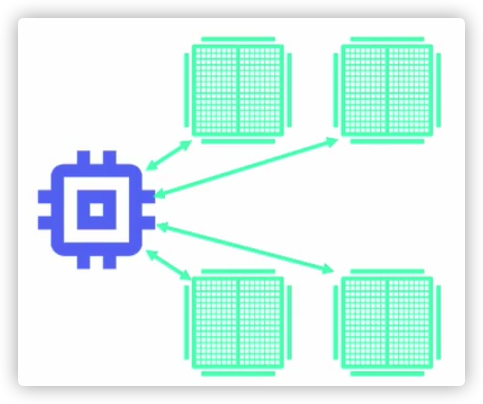``````central_storage_strategy = tf.distribute.experimental.CentralStorageStrategy()
``````

### 2.5 ParameterServerStrategy

TensorFlow 2 参数服务器使用异步方式来更新，即，会在各工作节点上独立进行变量的读取和更新，无需采取任何同步操作。因为工作节点彼此互不依赖，因此该策略可以对工作者进行容错处理，这样会在使用抢占式服务器时有所助益。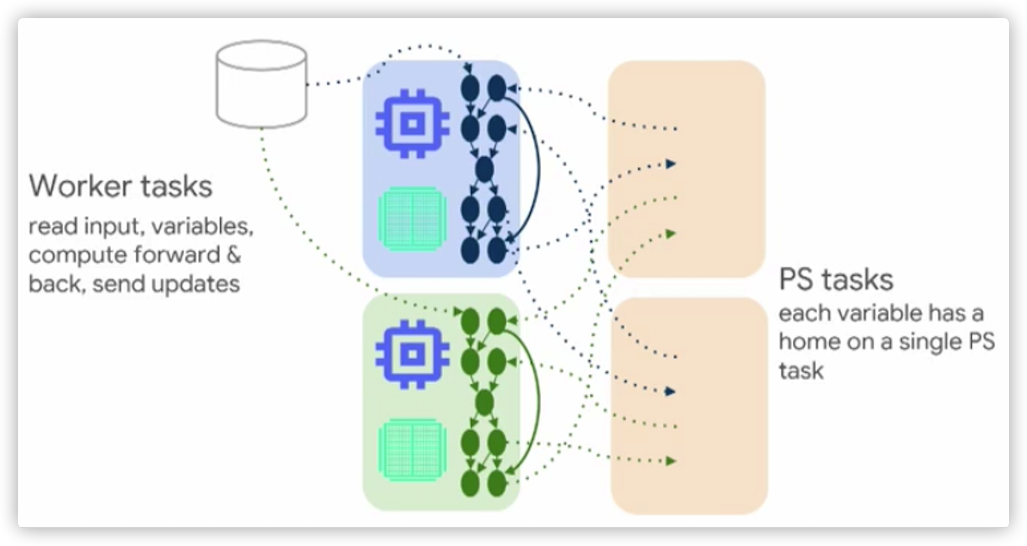``````strategy = tf.distribute.experimental.ParameterServerStrategy(
tf.distribute.cluster_resolver.TFConfigClusterResolver(),
variable_partitioner=variable_partitioner)
coordinator = tf.distribute.experimental.coordinator.ClusterCoordinator(
strategy)
``````

### 2.6 其他策略

#### 2.6.1 默认策略

``````default_strategy = tf.distribute.get_strategy()
``````

• 它允许无条件编写可感知分布的库代码。例如，在优化器中，我们可以执行 tf.distribute.get_strategy() 并使用该策略来规约梯度，而它将始终返回一个我们可以在其上调用 Strategy.reduce API 的策略对象。
``````# In optimizer or other library code
# Get currently active strategy
strategy = tf.distribute.get_strategy()
strategy.reduce("SUM", 1.)  # reduce some values
``````
• 与库代码类似，它可以使用户程序在使用或不使用分布策略的情况下都能工作，而无需条件逻辑。以下示例代码段展示了这一点：
``````if tf.config.list_physical_devices('gpu'):
strategy = tf.distribute.MirroredStrategy()
else:  # use default strategy
strategy = tf.distribute.get_strategy()

with strategy.scope():
# do something interesting
print(tf.Variable(1.))
``````

#### 2.6.2 OneDeviceStrategy

Tf.distribute.OneDeviceStrategy 是一种会将所有变量和计算放在单个指定设备上的策略。

``````strategy = tf.distribute.OneDeviceStrategy(device="/gpu:0")
``````

## 3. 在tf.keras.Model.fit 中使用

1. 创建一个合适的 tf.distribute.Strategy 实例。
2. 将 Keras 模型、优化器和指标的创建转移到 strategy.scope 中。

``````mirrored_strategy = tf.distribute.MirroredStrategy()

with mirrored_strategy.scope():
model = tf.keras.Sequential([tf.keras.layers.Dense(1, input_shape=(1,))])

model.compile(loss='mse', optimizer='sgd')
``````

``````dataset = tf.data.Dataset.from_tensors(([1.], [1.])).repeat(100).batch(10)
model.fit(dataset, epochs=2)
model.evaluate(dataset)
``````

``````import numpy as np
inputs, targets = np.ones((100, 1)), np.ones((100, 1))
model.fit(inputs, targets, epochs=2, batch_size=10)
``````

``````# Compute global batch size using number of replicas.
BATCH_SIZE_PER_REPLICA = 5
global_batch_size = (BATCH_SIZE_PER_REPLICA *
mirrored_strategy.num_replicas_in_sync)
dataset = tf.data.Dataset.from_tensors(([1.], [1.])).repeat(100)
dataset = dataset.batch(global_batch_size)

LEARNING_RATES_BY_BATCH_SIZE = {5: 0.1, 10: 0.15}
learning_rate = LEARNING_RATES_BY_BATCH_SIZE[global_batch_size]
``````## 4. 在自定义训练循环中使用

``````with mirrored_strategy.scope():
model = tf.keras.Sequential([tf.keras.layers.Dense(1, input_shape=(1,))])
optimizer = tf.keras.optimizers.SGD()
``````

``````dataset = tf.data.Dataset.from_tensors(([1.], [1.])).repeat(100).batch(
global_batch_size)
dist_dataset = mirrored_strategy.experimental_distribute_dataset(dataset)
``````

``````loss_object = tf.keras.losses.BinaryCrossentropy(
from_logits=True,
reduction=tf.keras.losses.Reduction.NONE)

def compute_loss(labels, predictions):
per_example_loss = loss_object(labels, predictions)
return tf.nn.compute_average_loss(per_example_loss, global_batch_size=global_batch_size)

def train_step(inputs):
features, labels = inputs

with tf.GradientTape() as tape:
predictions = model(features, training=True)
loss = compute_loss(labels, predictions)

return loss

@tf.function
def distributed_train_step(dist_inputs):
per_replica_losses = mirrored_strategy.run(train_step, args=(dist_inputs,))
return mirrored_strategy.reduce(tf.distribute.ReduceOp.SUM, per_replica_losses,
axis=None)
``````

1. 我们使用了 tf.nn.compute_average_loss 来计算损失。tf.nn.compute_average_loss 将每个样本的损失相加，然后将总和除以 global_batch_size。这很重要，因为稍后在每个副本上计算出梯度后，会通过对它们求和使其跨副本进行聚合。
2. 我们使用了 tf.distribute.Strategy.reduceAPI 来聚合 tf.distribute.Strategy.run 返回的结果。tf.distribute.Strategy.run 会从策略中的每个本地副本返回结果。目前有多种方法使用该结果，比如可以 reduce 它们以获得聚合值。还可以通过执行 tf.distribute.Strategy.experimental_local_results 获得包含在结果中的值的列表，每个本地副本一个列表。
3. 当在一个分布策略作用域内调用 apply_gradients 时，它的行为会被修改。具体来说，在同步训练期间，在将梯度应用于每个并行实例之前，它会对梯度的所有副本求和（sum-over-all-replicas）。

``````for dist_inputs in dist_dataset:
print(distributed_train_step(dist_inputs))
``````

``````iterator = iter(dist_dataset)
for _ in range(10):
print(distributed_train_step(next(iterator)))
``````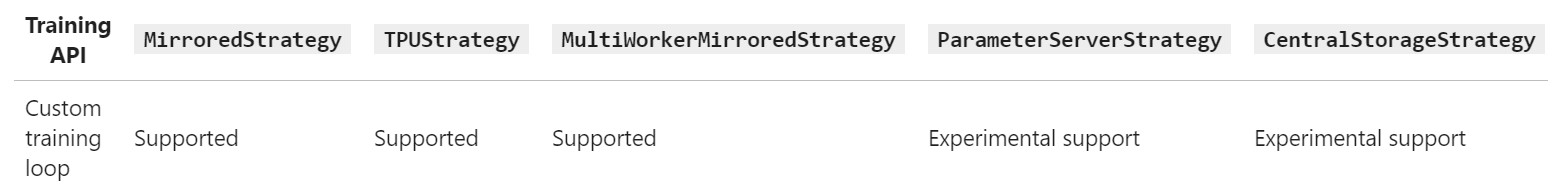## 5. 其他主题

### 5.1 设置 TF_CONFIG 环境变量

TF_CONFIG 有两个组件：cluster 和 task。

• cluster 会提供有关训练集群的信息，这是一个由不同类型的作业（如工作进程）组成的字典。在多工作进程训练中，通常会有一个工作进程除了要完成常规工作进程的工作之外，还要承担更多责任，如保存检查点和为 TensorBoard 编写摘要文件。此类工作进程称为 “chief” 工作进程，习惯上会将索引为 0 的工作进程指定为 chief 工作进程（实际上这是 tf.distribute.Strategy 的实现方式）。
• 另一方面，task 会提供有关当前任务的信息。第一个组件 cluster 对于所有工作进程都相同，而第二个组件 task 在每个工作进程上均不相同，并指定了该工作进程的类型和索引。

TF_CONFIG 的示例如下：

``````os.environ["TF_CONFIG"] = json.dumps({
"cluster": {
"worker": ["host1:port", "host2:port", "host3:port"],
"ps": ["host4:port", "host5:port"]
},
"task": {"type": "worker", "index": 1}
})
``````

## 0xFF 参考

https://github.com/tensorflow/docs-l10n/blob/master/site/en-snapshot/guide/distributed_training.ipynb

Tensorflow上手4: 初探分布式训练